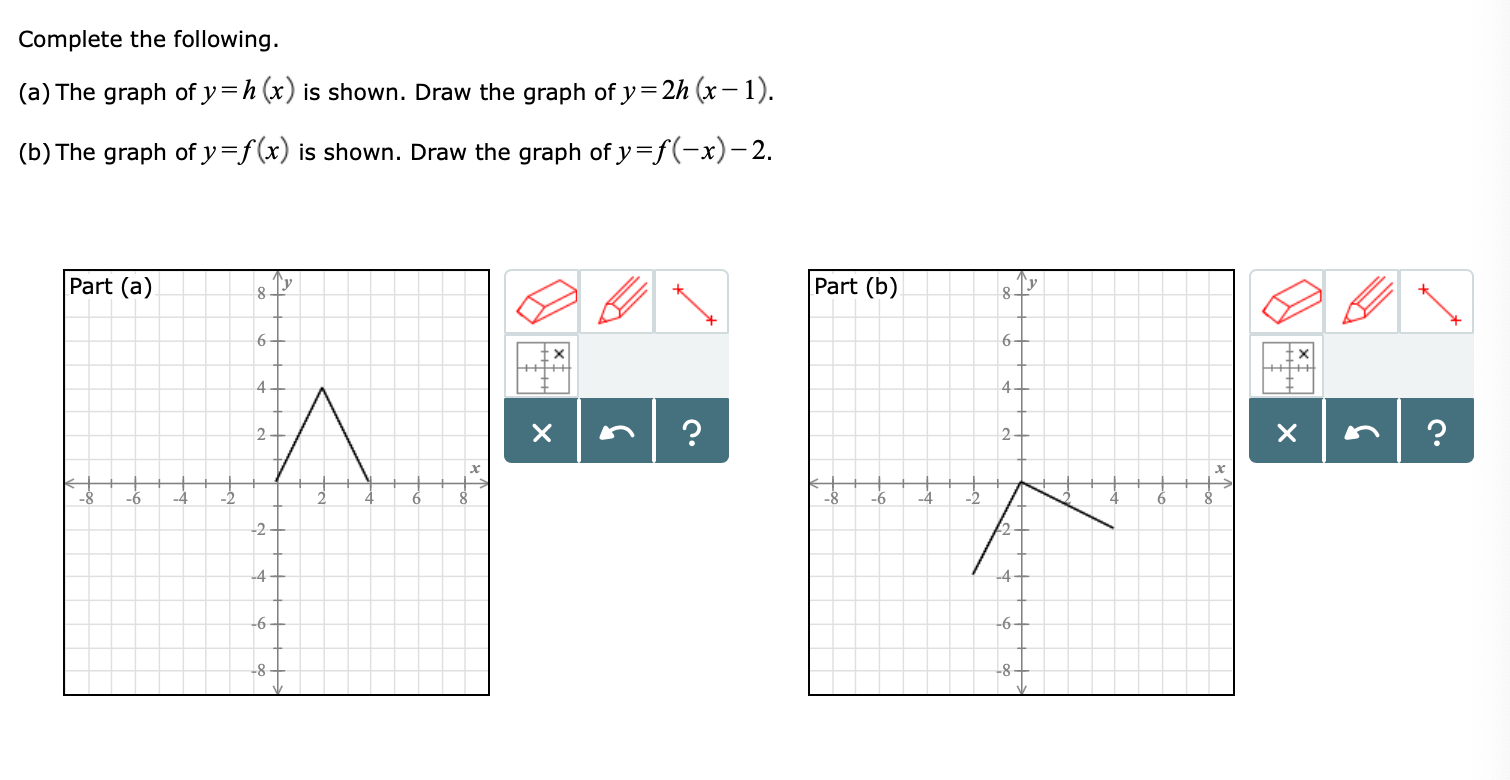# Complete the following.(a) The graph of y -h(x) is shown. Draw the graph of y-2h (x-1)(b) The graph ofy=f(x) is shown. Draw the graph ofy=f(-x)-2.Part (a)Part (b)-6

Question
66 views

I need help with part B please!help_outlineImage TranscriptioncloseComplete the following. (a) The graph of y -h(x) is shown. Draw the graph of y-2h (x-1) (b) The graph ofy=f(x) is shown. Draw the graph ofy=f(-x)-2. Part (a) Part (b) -6 fullscreen
check_circle

Step 1

Part (b):

To draw the graph of y = f(-x)-2, some of the following results has to be used.

Result used:

Horizontal Shifts

“Let f be a function and c a positive real number.

The graph of y = f (x + c) is the graph of y = f (x) shifted to the left c units.

The graph of y = f (x − c) is the graph of y = f (x) shifted to the right c units”.

Vertical Shifts

“Let f  be a function and c a positive real number.

The graph of y = f (x) + c is the graph of y = f (x) shifted c units vertically upward.

The graph of y = f (x) −c is the graph of y = f (x) shifted c units vertic...

### Want to see the full answer?

See Solution

#### Want to see this answer and more?

Solutions are written by subject experts who are available 24/7. Questions are typically answered within 1 hour.*

See Solution
*Response times may vary by subject and question.
Tagged in

### Math